# On the Beach

Nick finds thirty brown shells and twelve pink shells on the beach. Kim finds twenty-two brown shells and some pink shells on the beach. Kim shows her shells to Nick. Nick says, "We have the same number of shells!" How many pink shells does Kim find on the beach? Show all your mathematical thinking.

Assessment

## Plan

#### Formal Mathematical Language and Symbolic Notation

A student may independently select a printed number line, number chart, ten frames, graph paper, etc. as they work on a task.

### Suggested materials

Engagement Image:

Teachers may project the image below to launch this task for their students, define nouns, promote discussion, access prior knowledge, and inspire engagement and problem solving.

## Solutions tabs

### Expert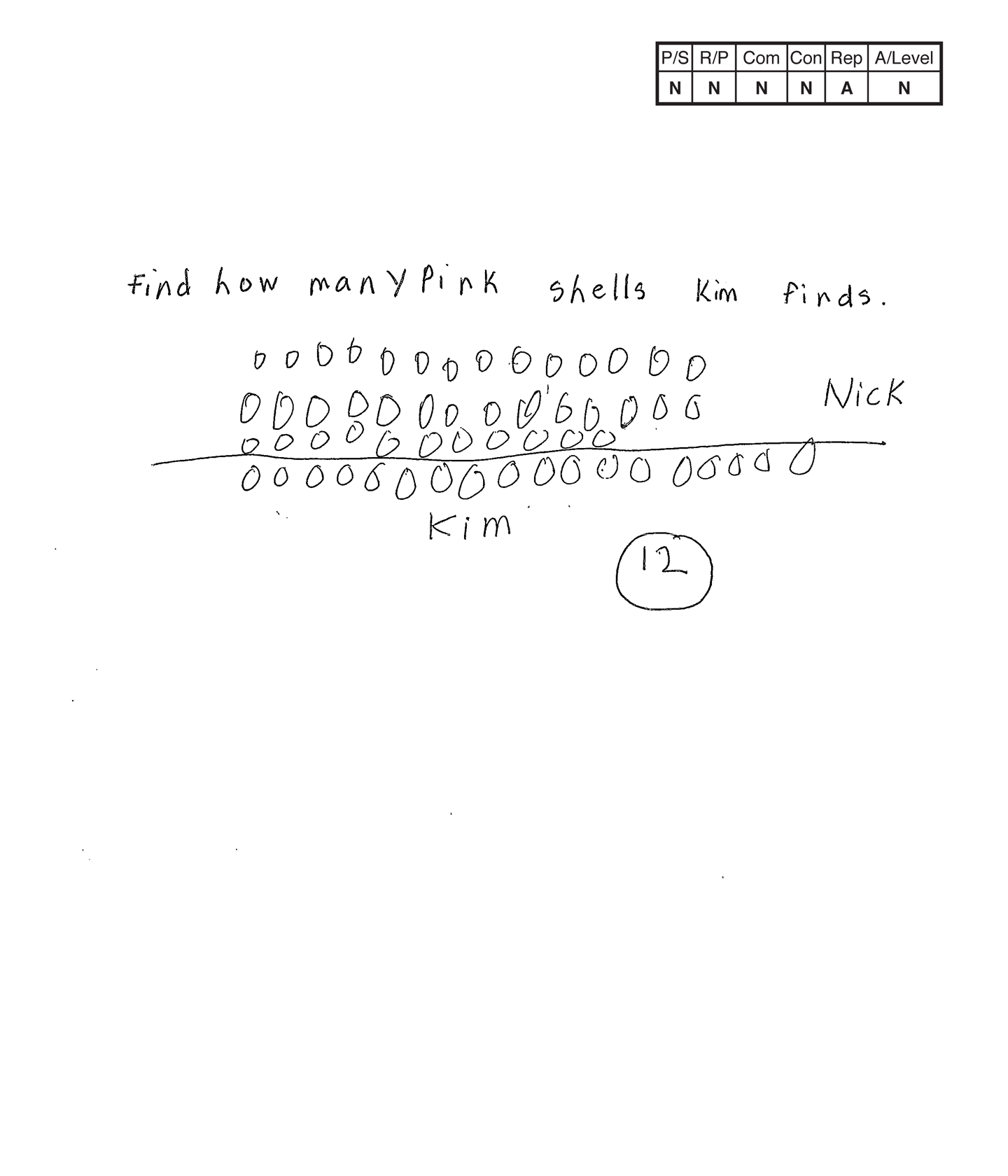This student does not meet the standard.

### Scoring Rationale

#### Novice

The student's strategy of diagramming thirty-nine circles for Nick and eighteen circles for Kim does not work to solve the task. The student's answer,"12," is not correct.

#### Novice

No correct reasoning is evident. A key does not define the O and the number of circles does not match the number of shells found by Nick and Kim.

#### Novice

The student does not use any mathematical terms.

#### Novice

The student does not attempt a mathematically relevant connection.

#### Apprentice

The student attempts a diagram. It appears that the circles represent shells and the student divides them between Nick and Kim. The number of circles does not match the numbers in a correct solution and no key was used to define the circle.

#### Novice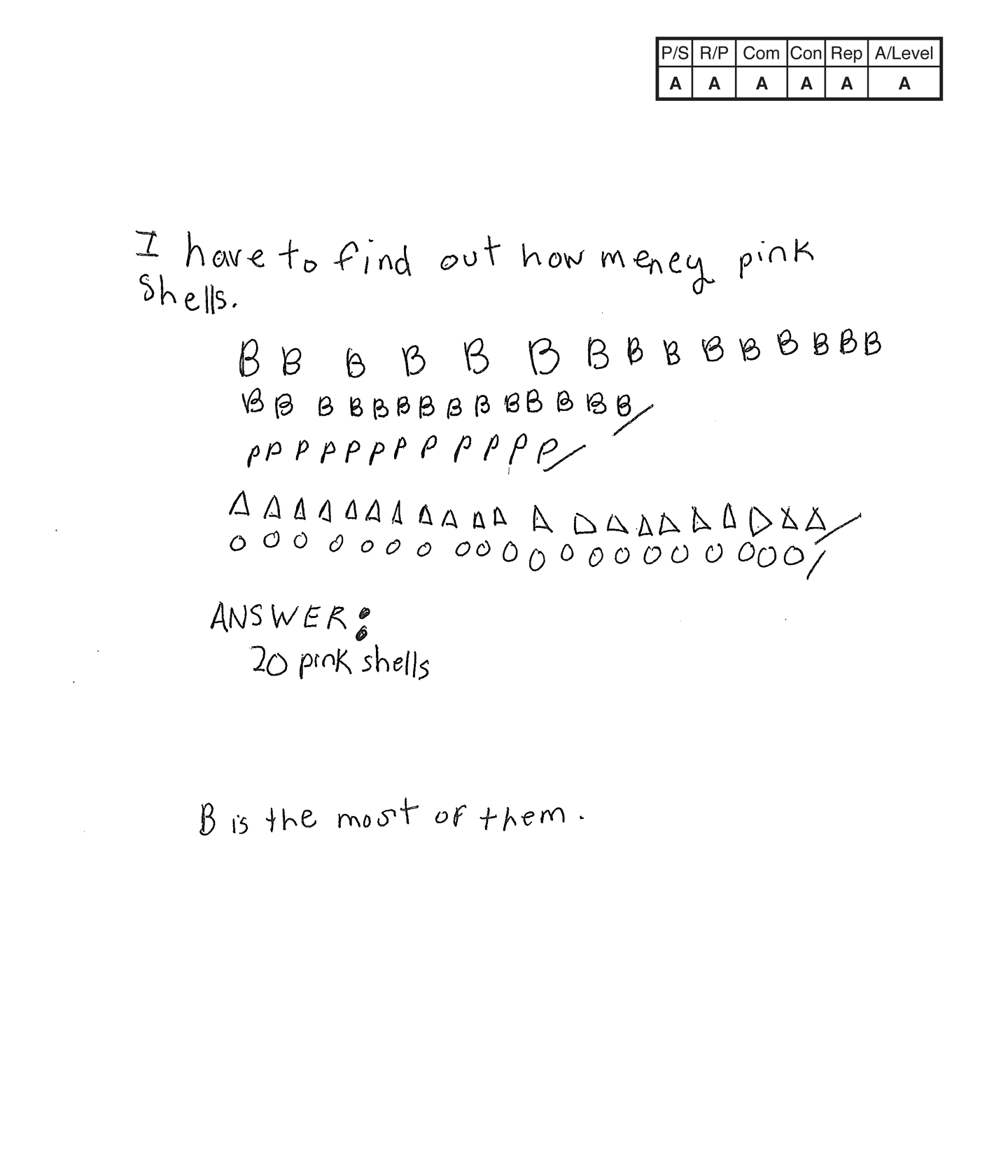This student does not meet the standard.

### Scoring Rationale

#### Apprentice

The student's strategy of diagramming the brown and pink shells for Nick and Kim would work to solve the task, but the student diagrams only twenty-nine brown shells for Nick and twenty-one brown shells for Kim and does not provide a key. The student's answer, "20 pink shells," is not supported by the student's diagram and is not considered correct.

#### Apprentice

Some correct reasoning is present. The student omits a key to define the B, P, △, O so one has to fill in a number of gaps to determine if the student is applying correct reasoning to the task.

#### Apprentice

The student correctly uses the mathematical term most.

#### Apprentice

The student attempts the mathematically relevant observation, "B is the most of them." The student never identifies that B represents brown shells so does not achieve the Practitioner performance level.

#### Apprentice

The student's diagram of the brown and pink shells for Kim is appropriate to the problem but not accurate. A key is not provided to identify each color of shell. It appears that one brown shell for Nick and one brown shell for Kim is missing.

#### Apprentice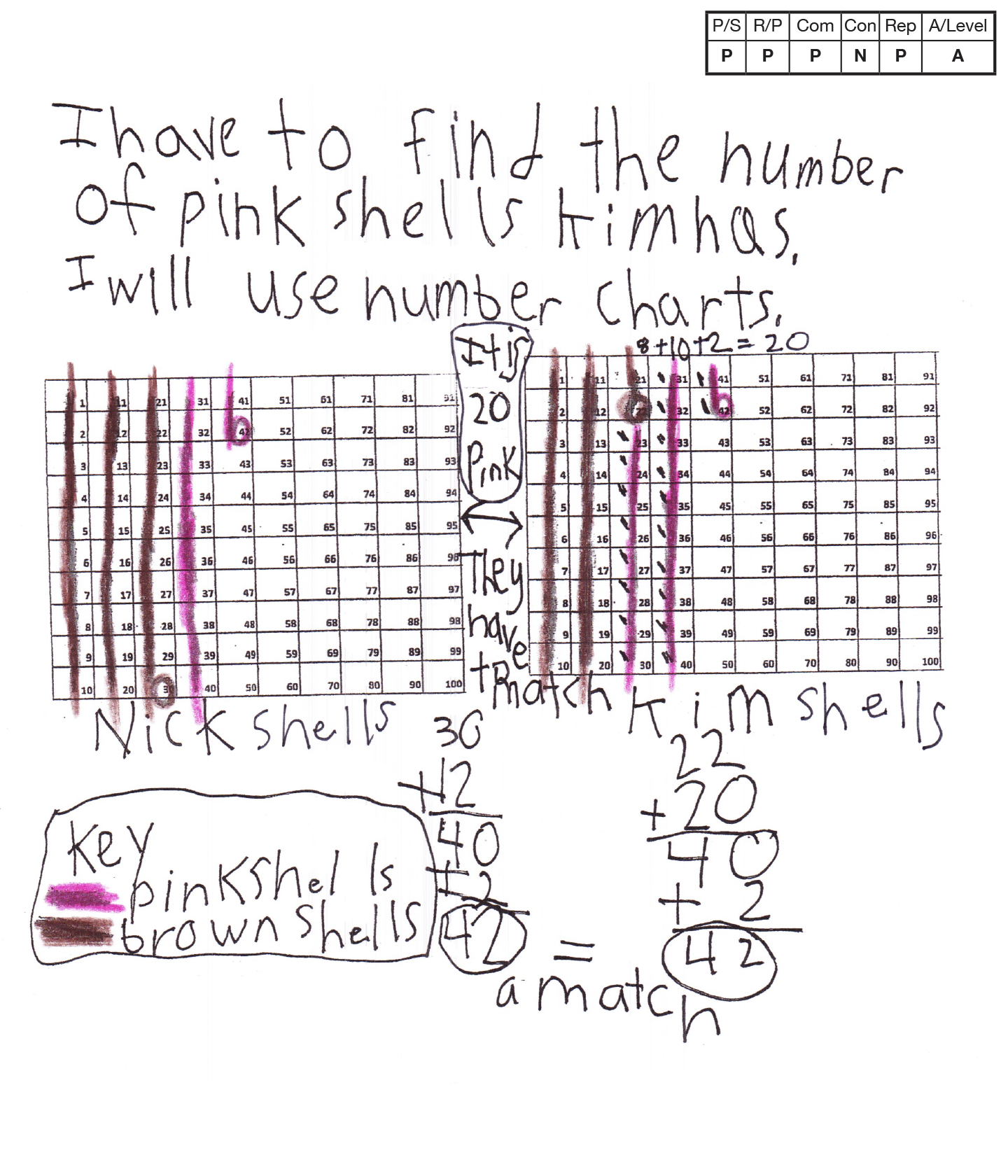This student does not meet the standard.

### Scoring Rationale

#### Practitioner

The student's strategy of using two number charts to indicate the number of brown and pink shells for Nick and Kim works to solve the task. The student's answer, "It is 20 pink," is correct.

#### Practitioner

The student's solution is constructed with adequate mathematical basis. The student demonstrates understanding of the underlying concepts of addition and comparing sums. The student also uses one-to-one correspondence when finding the total number of Kim's pink shells.

#### Practitioner

The student correctly uses the mathematical term—number from the task. The student also correctly uses the terms number charts and key.

#### Novice

The student solves the task and stops without making a mathematically relevant observation about their solution. The student has to know there are 42 brown and pink shells to find a correct answer.

#### Practitioner

The student's number charts are appropriate to the task and accurate. The student labels each chart. A key defines the pink and brown lines as pink and brown shells.

#### Apprentice

Note:

The overall achievement level for this piece of student work falls under Exemplars exception to the rule category. If a student has all Apprentice scores or above, but a Novice in "Connections," the student may still receive an achievement level score of Apprentice. To learn more about Exemplars scoring, please refer to the section of your dashboard called "Tools for Success" and click on the link for “Using the Assessment Rubric.”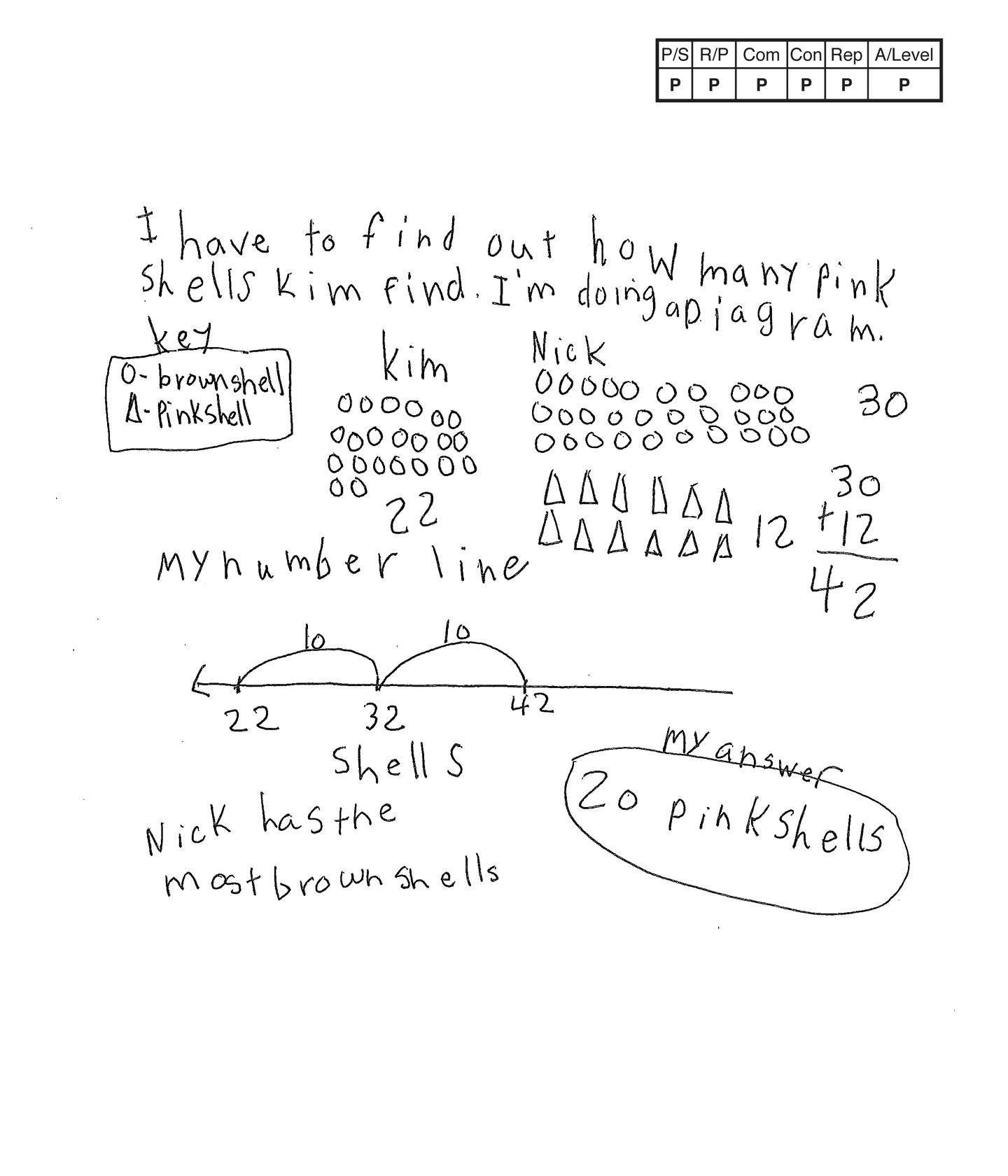This student meets the standard.

### Scoring Rationale

#### Practitioner

The student's strategy of diagramming Nick's brown and pink shells for forty-two shells and twenty brown shells for twenty-two shells, and then determining that Kim has twenty pink shells, works to solve the task. The student's answer, "20 pink shells," is correct.

#### Practitioner

The student's solution is constructed with adequate mathematical basis. The student demonstrates understanding of the underlying concepts of addition and subtraction to find results unknown.

#### Practitioner

The student correctly uses the mathematical terms diagram, key, number line, most.

#### Practitioner

The student makes the mathematically relevant observation, "Nick has the most brown shells."

#### Practitioner

The student's diagram of the brown shells for Kim and Nick and the pink shells for Nick is appropriate to the task and accurate. A key defines the brown and pink shells. The student's number line is appropriate and accurate with the shells labeled.

#### Practitioner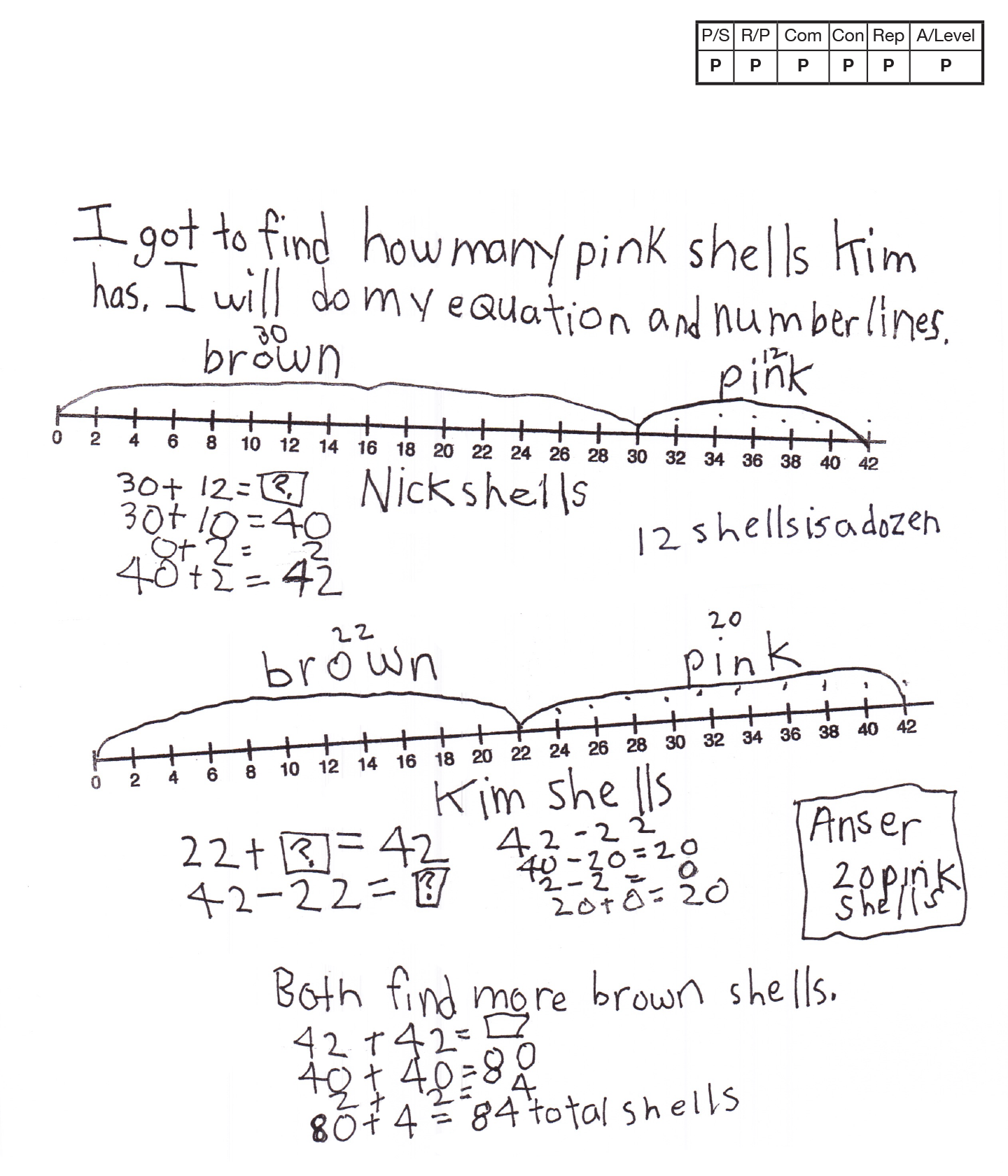This student meets the standard.

### Scoring Rationale

#### Practitioner

The student's strategy of using number lines to indicate Nick's brown and pink shells, Kim's brown and pink shells, and applying understanding of finding unknown quantities works to solve the task. The student's answer, "20 pink shells," is correct.

#### Practitioner

The student's solution is constructed with adequate mathematical basis. The student demonstrates understanding of the underlying concepts of addition and subtraction to find unknown quantities and comparison of two totals.

#### Practitioner

The student correctly uses the mathematical terms—equation, number lines, dozen, more and total.

#### Practitioner

The student makes mathematically relevant observations. The student states "12 shells is a dozen," "Both find more brown shells," and "80 + 4 = 84 total shells."

#### Practitioner

The student's number lines of the amount of brown and pink shells Nick and Kim each have is appropriate to the task and accurate. Each number line is labeled and the "jumps" are indicated.

#### Practitioner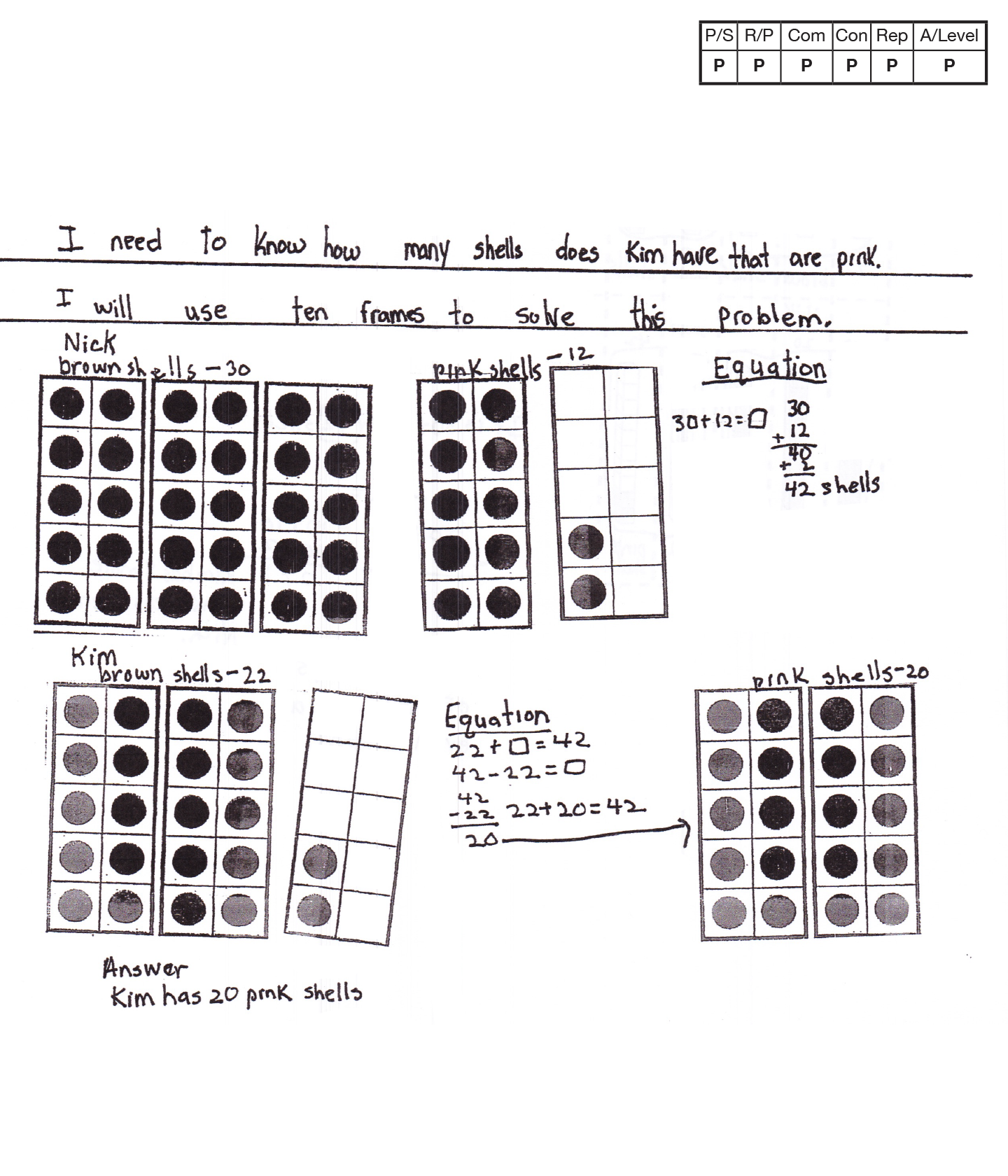,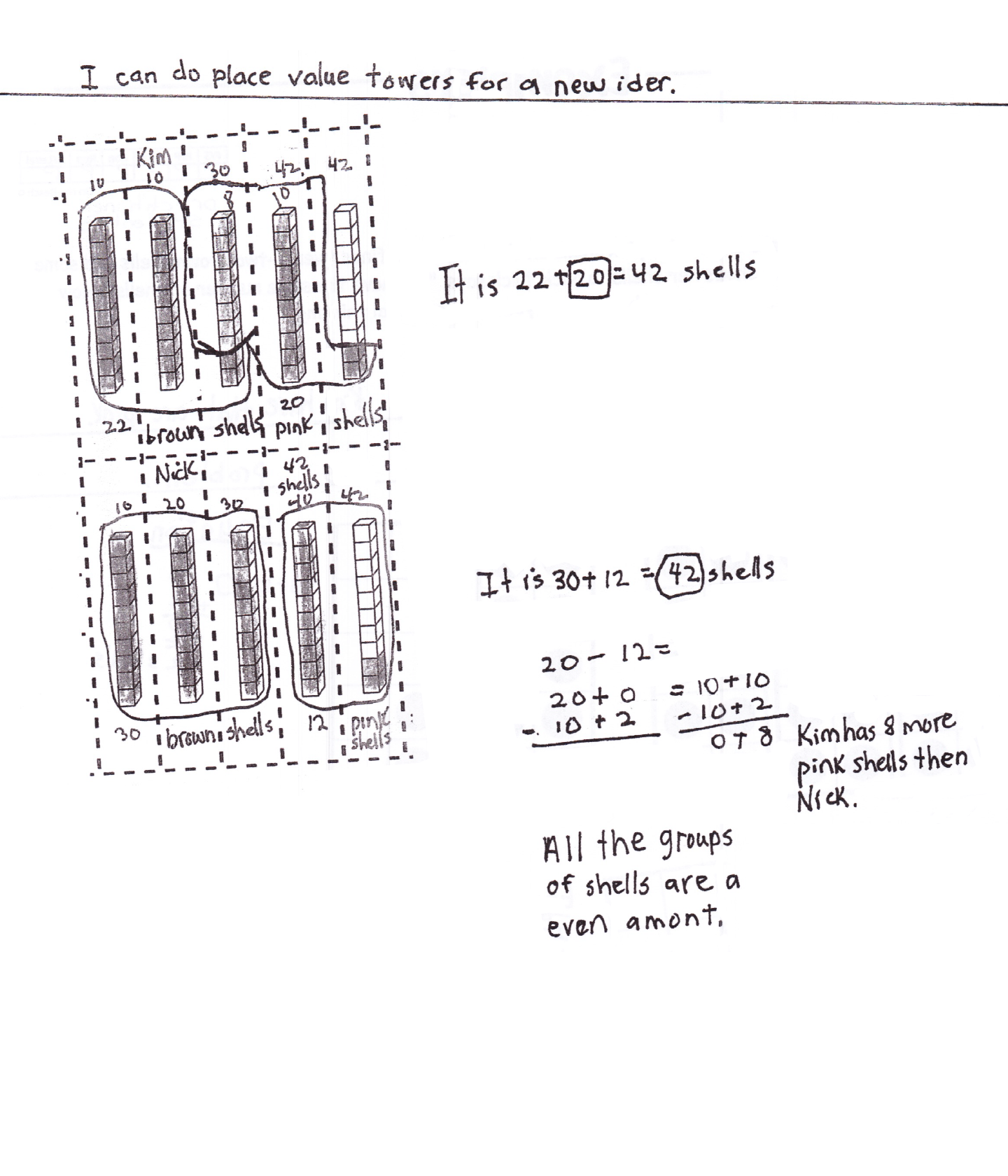This student meets the standard.

### Scoring Rationale

#### Practitioner

The student's strategy of using ten frames to represent the number of brown and pink shells Nick and Kim each find on the beach works to solve the task. The student's answer, "Kim has 20 pink shells," is correct.

#### Practitioner

The student's solution is constructed with adequate mathematical basis. The student demonstrates understanding of the underlying concepts of addition, subtraction, and using equations with unknown quantities.

#### Practitioner

The student correctly uses the mathematical terms—ten frames, equation, place value, more, even, "amont," and groups.

#### Practitioner

The student makes mathematically relevant connections. The student uses place value strips to represent the number of brown and pink shells Kim and Nick have. The student states, "Kim has 8 more pink shells then Nick," and "All the groups of shells are an even amont."

#### Practitioner

The student's ten frames are appropriate and accurate. All necessary labels are provided and the "dots" are correct. The student's place value strips are appropriate to the task and accurate. All necessary labels are provided and the correct amount of "shells" are highlighted.

#### Practitioner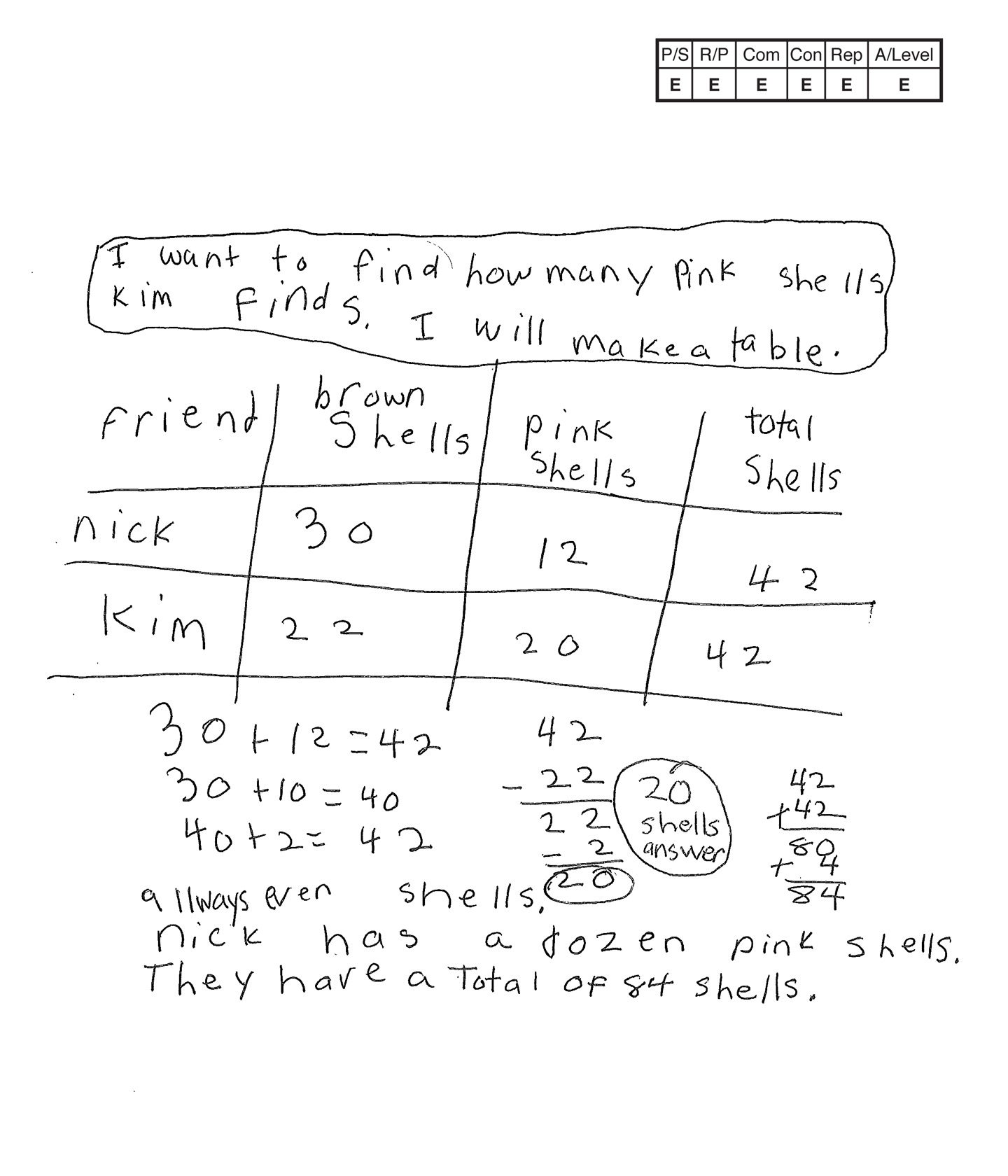,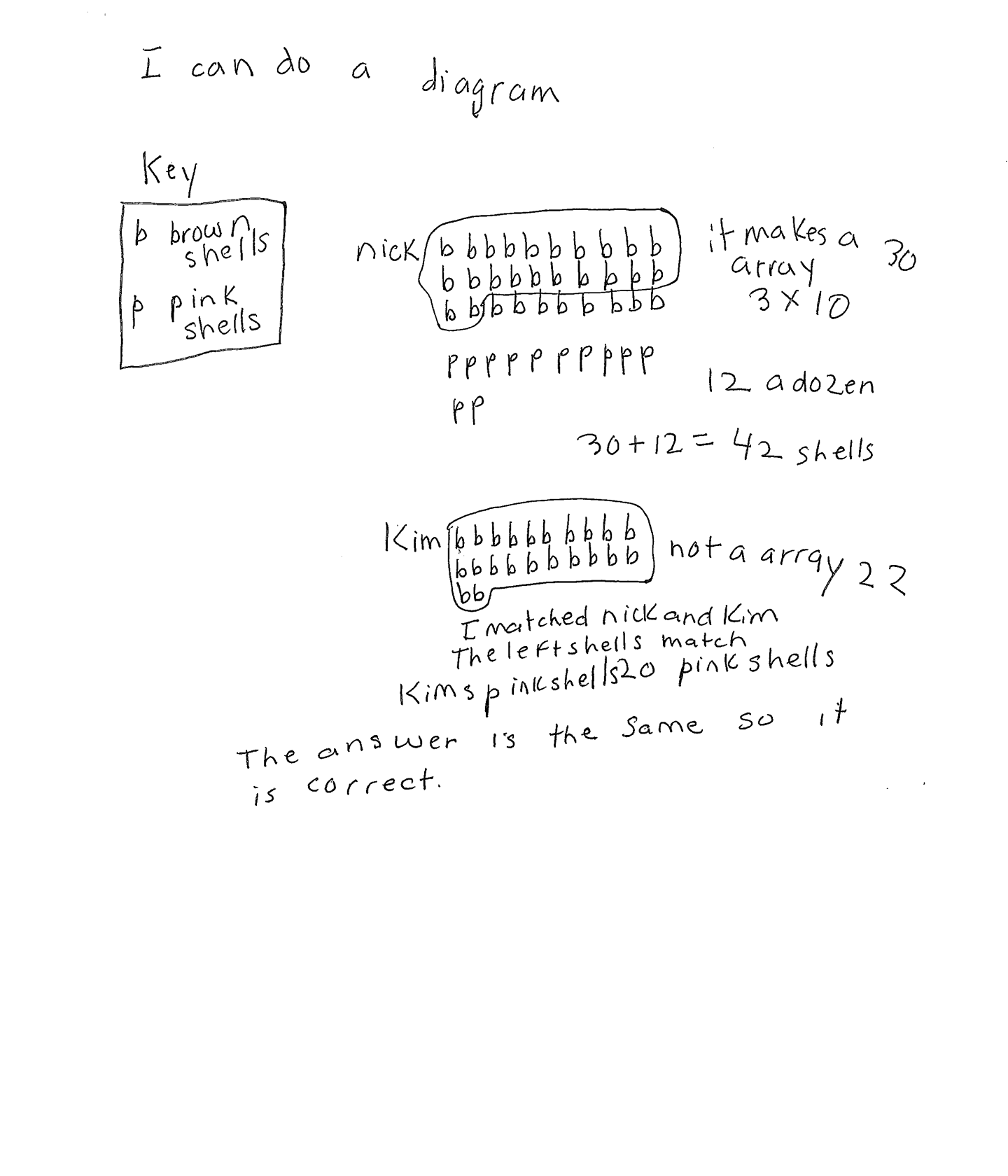This student exceeds the standard.

### Scoring Rationale

#### Expert

The student's strategy of creating a table to record the number of brown and pink shells Nick and Kim find on the beach works to solve the task. The student's answer, "20," is correct. The student verifies their answer with a diagram.

#### Expert

The student's solution is constructed with adequate mathematical basis. The student demonstrates understanding of the underlying concepts of addition and subtraction to find results unknown. The student uses a diagram to verify their answer.

#### Expert

The student correctly uses the mathematical terms table, total, even, dozen, diagram, key, array. The student uses the mathematical notation 3 x 10, (3 by 10).

#### Expert

The student makes the mathematically relevant Practitioner connections, "allways even shells," "Nick has a dozen pink shells," and, "They have a total of 84 shells." The student makes the Expert connection by using a diagram to verify that their answer is correct. The student states, "The answer is the same so it is correct."

#### Expert

The student's table is appropriate and accurate. All labels are provided and the data is correct. The student uses a diagram to verify their answer. The diagram is accurate and a key defines the brown and pink shells.